## NCERT Solutions for Class 11 Physics Chapter 13 Kinetic Theory

These Solutions are part of NCERT Solutions for Class 11 Physics. Here we have given NCERT Solutions for Class 11 Physics Chapter 13 Kinetic Theory

Question 1.
Estimate the fraction of molecular volume to the actual volume occupied by oxygen gas at STP. Take the diameter of an oxygen moleculer to be roughly 3 A.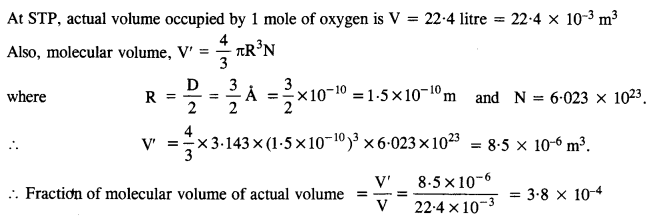Question 2.
Molar volqme is the volume occupied by 1 mol of any (ideal) gas at standard temperature and pressure (STP : 1 atmospheric pressure, 0°C). Show that it is 22.4 litres.
For one mole of an ideal gas, we have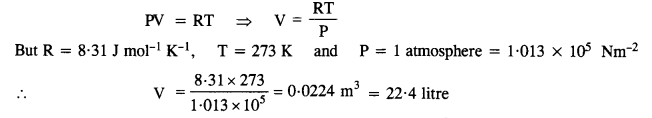Question 3.
Following figure shows plot of PV/T versus P for 1.00 x 10-3 kg of oxygen gas at two different temperatures.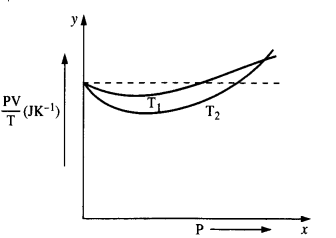(a) What does the dotted plot signify ?
(b) What is true : Tx > T2 or Tx < T2 ? ‘
(c) What is the value of PV/T where the curves meet on the y-axis ?
(d) If we obtained similar plots for 1.00 X 10-3 kg of hydrogen, would we get the same value of $$\frac { PV}{ T }$$ at the point where the curves meet on the y-axis ? If not, what mass of hydrogen yields the same value of $$\frac { PV}{ T }$$ (for low pressure-high temperature region of the plot) ?
(Molecular mass of H2 = 2.02 u, of O2 = 32.0 u, R = 8.31 mol-1 K_1).
(a) The dotted plot shows that is a constant quantity $$\frac { PV}{ T }$$ . This signifies the ideal gas behaviour.
(b) Here T1 > T2. This is because curve at T1 is close to ideal behaviour of gas which occurs at higher temperature.
(c) At the point where the curve meets the y-axis, we have $$\frac { pv}{ T }$$ = μR, where p is the number of moles of oxygen gas.
Here, Mass of oxygen, m = 1.00 x 10-3 kg
Also, molecular mass, M = 32 X 10-3 kg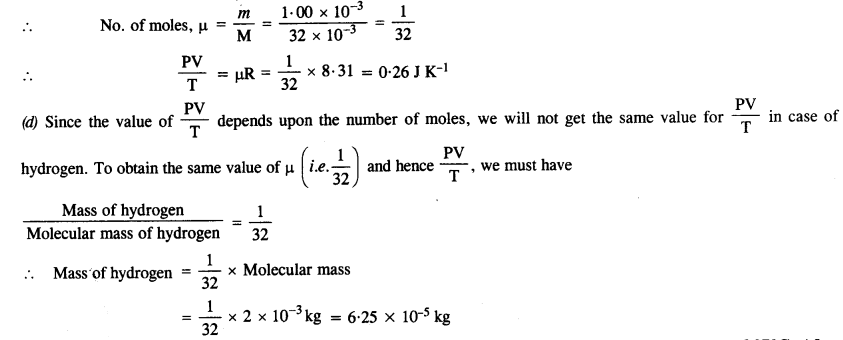Question 4.
An oxygen cylinder of volume 30 liters has an initial gauge pressure of 15 atm and a temperature of 27°C. After some oxygen is withdrawn from the cylinder, the gauge pressure drops to 11 atm and its temperature drop to 17°C. Estimate the mass of oxygen taken out of the cylinder. (R = 8.31 J mol-1 K_1, molecular mass of O2 = 32 u).
Under the initial conditions,
V = 30 liter = 30 x 10-3 m-3, P = 15 atm = 15 x 1.01 x 105 Pa
T = 27 °C = 273 + 27 = 300 K.
Also, R = 8.31 J mol-1 K-1 and molar mass, M = 32 x 10″3 kg.
∴ Using the relation PV = μRTQuestion 5.
An air bubble of volume 1.0 cm3 rises from the bottom of a lake 40 m deep at a temperature of 12°C. To what volume does it grow when it reaches the surface, which is at a temperature of 35 °C ?
Volume of the bubble inside, V1 = 1.0 cm3 = 1 x 10-6 m3
Pressure on the bubble, P1 = Pressure of water 4- Atmospheric pressure
= ρgh + 1.01 x 105 = 1000 x 9.8 x 40 + 1.01 x 105
= 3.92 x 105 + 1.01 x 105 = 4.93 x 105 Pa
Temperature, T, = 12°C = 273 + 12 = 285 K
Also, the pressure outside the lake, P2 = 1.01 x 105 N m-2Question 6.
Estimate the total number of air molecules (inclusive of oxygen, nitrogen, water vapour and other constituents) in a room of capacity 25.0 m3 at a temperature of 27°C and 1 atm pressure.
Here, P = 1 atm = 1 x 1.01 x 105 Pa,
V = 25.0 m3, T = 27°C = 27 + 273 = 300 K and
R = 8.31 J mol-1 K-1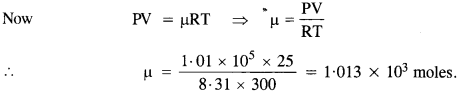Now, one mole of a gas contains = 6.023 x 1023 molecules
1.013 x 103 moles would contain = 6.023 x 1023 x 1.013 x 103
= 6.10 x 1026 molecules.
= 6.10 x 1026 molecules.

Question 7.
Estimate the average thermal energy of a helium atom at
(1) room temperature (27°C),
(2) the temperature on the surface of the Sun (6000 K),
(3) the temperature of 10 million kelvin (the typical core temperature in the case of a star).Question 8.
Three vessels of equal capacity have gases at the same temperature and pressure. The first-vessel contains neon (monoatomic), the second contains chlorine (diatomic), and the third contains uranium hexafluoride (polyatomic). Do the vessels contain an equal number of respective molecules? Is the root mean square speed of molecules the same in the three cases? If not, in which case is υrms the largest?
Equal volumes of all the gases under similar conditions of pressure and temperature contain an equal number of molecules (according to Avogadro’s hypothesis). Therefore, the number of molecules in each case is the same.
The rms velocity of molecules is given by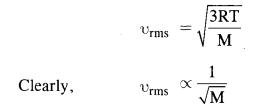Since Neon has minimum atomic mass M, its rms velocity is maximum.

Question 9.
At what temperature is the root mean square speed of an atom in an argon gas cylinder equal to the rms speed of a helium gas atom at – 20°C? (atomic mass of Ar = 39.9 u, of He = 4.0 u).
For argon, atomic mass m  = 39.9 u = 39.9 X 1.67 x 10-27 kg = 6.66 x 10-26 kgQuestion 10.
Estimate the mean free path and collision frequency of a nitrogen molecule in a cylinder containing nitrogen at 2.0 atm and temperature 17°C. Take the radius of a nitrogen molecule to be roughly 1.0 A. Compare the collision time with the time the molecule moves freely between two successive collisions (Molecular mass of N2 = 28.0 u).Question 11.
A meter-long narrow bore held horizontally (and closed at one end) contains a 76 cm long mercury thread, which traps a 15 cm column of air. What happens if the tube is held vertically with the open end at the bottom?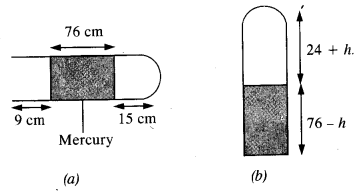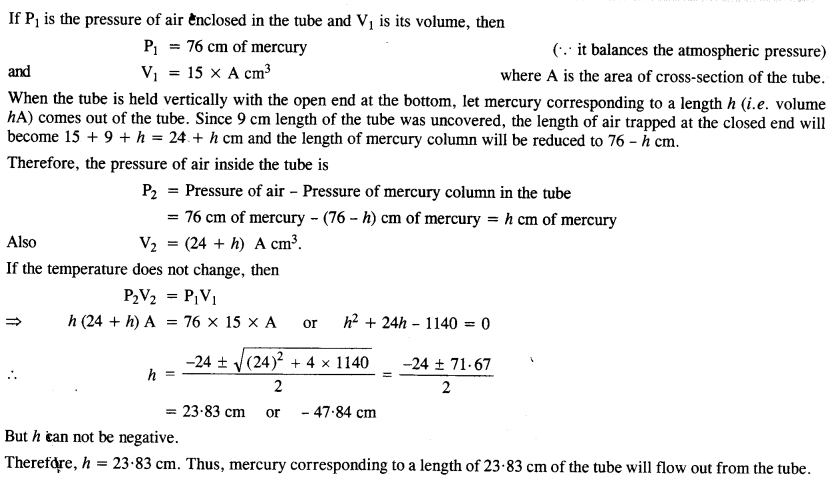Question 12.
From ascertain apparatus, the diffusion rate of hydrogen has an average value of 28.7 cm3 s-1. The diffusion of another gas under the same conditions is measured to have an average rate of 7.2 cm3 s-1. Identify the gas.
Here, r1 = 28.7 cm3 s_1, r2 = 7.2 cm3 s-1, M1 = 2g and M2 = ?
∴ Using Graham’s law of diffusion,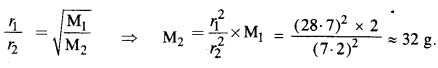This is the molecular mass of oxygen. Therefore, the other gas is oxygen

Question 13.
A gas in equilibrium has uniform density and pressure throughout its volume. This is strictly true only if there are no external influences. A gas column under gravity, for example, does not have a uniform density (and pressure). As you might expect, its density decreases with height. The precise dependence is given by the so-called law of atmosphereswhere n2, n1 refer to number density at heights h2 and hx
respectively. Use this relation to derive the equation for sedimentation equilibrium of a suspension in a liquid columnwhere ρ is the density of the suspended particle, and ρ’ that of the surrounding medium. [NA is Avogadro’s number, and R the universal gas constant].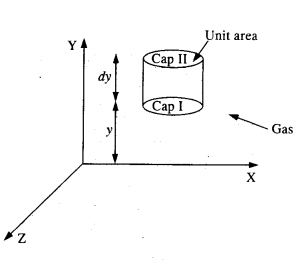Consider the molecules of a gas uniformly distributed in a given region of space. Let p be die density of the gas and it is in thermodynamical equilibrium.

Consider an imaginary cylinder of this gas having a unit area of cross-section and placed vertically. Let Y direction be the vertical direction so that the lower cap I of the cylinder is parallel to XZ plane at a height y + dy above the XZ plane as shown in Fig. Let P and P + dP be the pressures at the caps I and cap II respectively

Then the force acting on the cap I due to gravity is the weight of cylinder
W = Mass of cylinder X g = Vρg = 1 X dy pg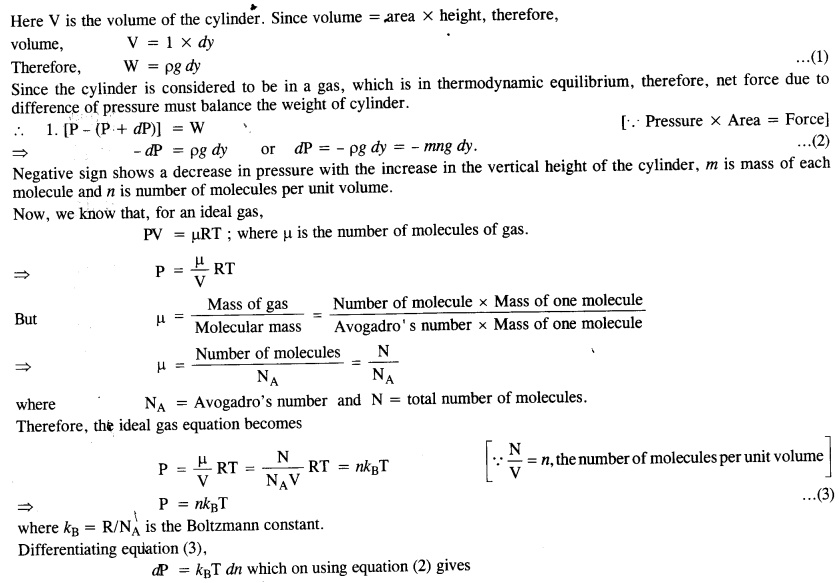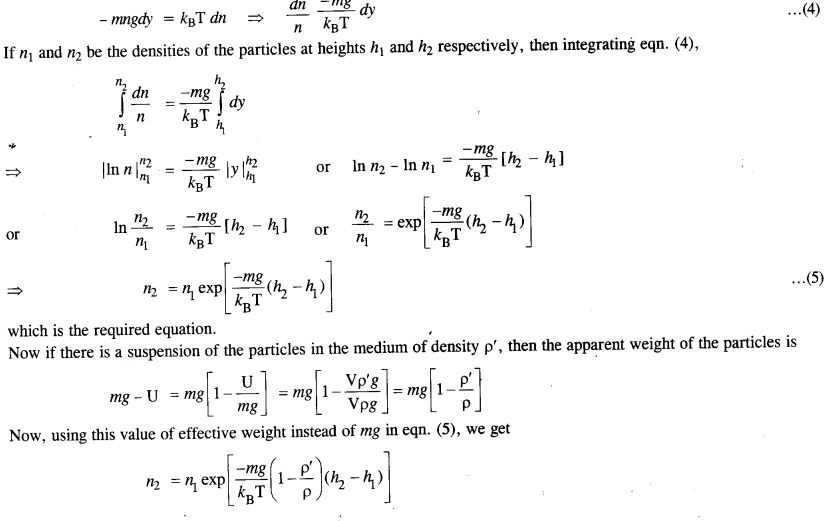Question 14.
Given below are densities of some solids and liquids. Give rough estimates of the size of their atoms :

 Substance Atomic mass Density (103 kg m3-3)] Carbon (diamond) 12.01 2.22 Gold 197.0 19.32 Nitrogen (Liquid) 14.01 1.00 Lithium 6.94 0.53 Fluorine (liquid) 19.00 1.14